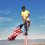# Motion in Gravitational Fields

I would like to share some insight on how different trajectories can form in a central field. I'll use laws of Newtonian gravitation and I would limit myself to examples in gravitation.

#### Two Body Central Force

In a two body system, we normally assume the heavier to be fixed. I will not. Also, I will proceed by taking the motion of the reduced mass of the system.Going down to basic equations, coordinate of C.M. becomes $\vec R = \dfrac{m_1\vec r_1 + m_2\vec r_2}{M}$ where $M = m_1 + m_2$. Now, using $\vec r = \vec r_1 - \vec r_2$, $\vec r_1 = \vec R + \dfrac{m_2}M \vec r\\\vec r_2 = \vec R - \dfrac{m_1}M \vec r$

Note that the momentum $\vec P = M\dot {\vec R} = 0$ implies that the centre of mass is stationary ($\vec R = 0$)

Looking at Kinetic Energy, \begin{aligned}T &= \dfrac12\left(m_1\dot r_1^2+m_2\dot r_2^2\right)\\ &=\dfrac12\left[m_1\left(\dot R + \dfrac{m_2}M \dot r\right)^2+m_1\left(\dot R - \dfrac{m_1}M \dot r\right)^2\right]\\ &=\dfrac12 M\dot R^2+\dfrac12 \mu\dot r^2 \end{aligned} where $\mu = \dfrac{m_1m_2}M$, the reduced mass of the system. It's time for us to shift to the centre of mass frame of reference.

## The Centre of Mass reference frame

From now on, all standard variables will be referred on the C.M. frame.

In C.M. frame, for example, our kinetic energy becomes, $T = \dfrac12 \mu\dot r^2$.

#### Equation of Motion

Let our central force and it's potential be $\vec F = -f(r)\ \hat r\\U = U(r)$

The angular momentum of the system (w.r.t. CM) will become, $\vec L = \left(m_1r_1^2+m_2r_2^2\right)\omega = \mu r^2 \dot \theta$

Using $\dot{\vec r} = \dot r \ \hat r + r\dot\theta\ \hat\theta$, $T = \dfrac12 \mu\left(\dot r^2 + r^2\dot\theta^2\right)$

Thus, the total energy, \begin{aligned} E &= T+U\\ &= \dfrac12 \mu\left(\dot r^2 + r^2\dot\theta^2\right) + U(r)\\ &= \dfrac12 \mu\dot r^2 + \dfrac{L^2}{2\mu r^2} + U(r)\\ E&= \dfrac12 \mu\dot r^2 + U_{\text{eff}}\end{aligned} where $U_{\text{eff}} = \dfrac{L^2}{2\mu r^2} + U(r)$

Note: I've written rotational kinetic energy in terms of $L$ is because $\theta$ term is messy and $L$ is constant.

This won't be updated. I'm tired, sorry.Note by Kishore S. Shenoy
3 years ago

This discussion board is a place to discuss our Daily Challenges and the math and science related to those challenges. Explanations are more than just a solution — they should explain the steps and thinking strategies that you used to obtain the solution. Comments should further the discussion of math and science.

When posting on Brilliant:

• Use the emojis to react to an explanation, whether you're congratulating a job well done , or just really confused .
• Ask specific questions about the challenge or the steps in somebody's explanation. Well-posed questions can add a lot to the discussion, but posting "I don't understand!" doesn't help anyone.
• Try to contribute something new to the discussion, whether it is an extension, generalization or other idea related to the challenge.

MarkdownAppears as
*italics* or _italics_ italics
**bold** or __bold__ bold
- bulleted- list
• bulleted
• list
1. numbered2. list
1. numbered
2. list
Note: you must add a full line of space before and after lists for them to show up correctly
paragraph 1paragraph 2

paragraph 1

paragraph 2

[example link](https://brilliant.org)example link
> This is a quote
This is a quote
    # I indented these lines
# 4 spaces, and now they show
# up as a code block.

print "hello world"
# I indented these lines
# 4 spaces, and now they show
# up as a code block.

print "hello world"
MathAppears as
Remember to wrap math in $$ ... $$ or $ ... $ to ensure proper formatting.
2 \times 3 $2 \times 3$
2^{34} $2^{34}$
a_{i-1} $a_{i-1}$
\frac{2}{3} $\frac{2}{3}$
\sqrt{2} $\sqrt{2}$
\sum_{i=1}^3 $\sum_{i=1}^3$
\sin \theta $\sin \theta$
\boxed{123} $\boxed{123}$

Sort by:

Great Work

- 3 years ago

Okay, this is dead. I have no idea what I intended to say after this. Good day!

- 2 months, 3 weeks ago